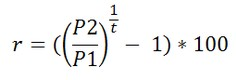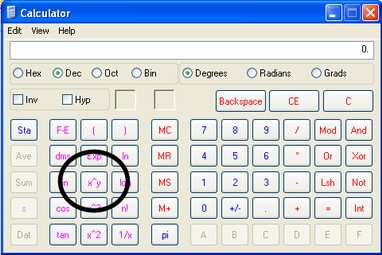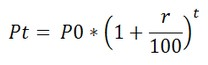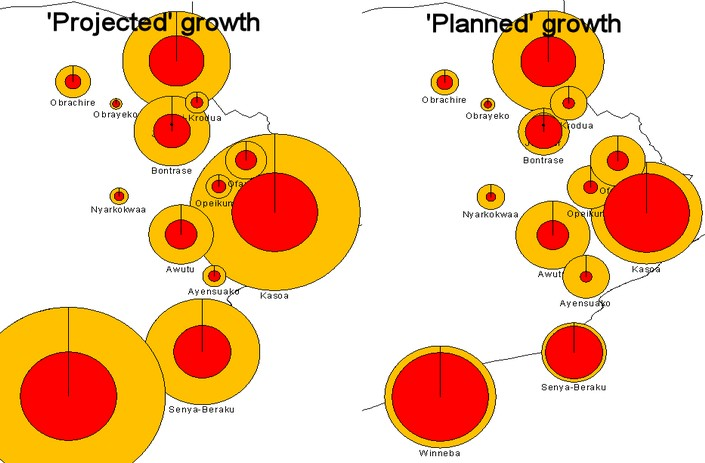# LUPMIS - GIS User Manual for Land Use Planning

## A15.1 Population projected and planned population forecast

Annexes > A15. Population statistics

Annex 15: Population Statistics

Level of expertise required for this Chapter: Advanced; specifically for LUPMIS @ TCPD

A15.1 Population Projection and Planned Population Forecast

The 'Printing Tools' have a 'Population Diagram' option, which enables an automatic outprint of population figures, displayed as circles on the map; see Chapter 6.10.4 for its documentation.

The '
Census Tools' have a utility 'Growth rate' for these calculations.

1. Growth rate:

Based on two population figures of the same community of different years, you can calculate the growth rate, with:

• t: Number of years of census period, for example from 1984-2000: 16
• P2: Population at the end of the census period, for example 200000
• P1: Population at the start of the census period, for example 150000
• r: Growth annual rate (in %)

The formula is:Step Calculate Example 1.1 1 / t 1/16 = 0.0625 1.2 P2 / P1 200000/150000 = 1.3333 1.3 Result of step 1.2 to the power of result of step 1.1 (*) 1.3333 to the power of 0.0625 = 1.0181 1.4 Result of step 1.3 - 1, * 100 (to have the result in %) 1.0181 - 1 = 0.0181 --> 1.81 %

(*) How to calculate 'to the power of':
With Windows calculator, you have to turn on the scientific calculator by
View > Scientific. Then: Enter base number > x^y > power number > = .
In Excel: You use the = POWER(a,b) function, where a = base number, b = exponent (see next Annex 15.2).For comparison: Urban growth rate in Ghana from 1984-2000 is calculated as 4.2% p.a. The city of Accra had a reported growth rate from 1984-2000 of 3.4% p.a., city of Kumasi of 5.5%, Kasoa of 17.6% (Sources: UN and www.citypopulation.de).

2. Population projection:

With this calculated growth rate and the assumption of continouing growth at the same pace, you can project the future population.

• t: Number of years, for example 5 years
• Pt: Population after t years
• P0: Population at the start, for example 200000
• r: Growth annual rate (in %), for example 1.81

The formula is:Step Calculate Example 2.1 1 + r / 100 1 + 1.81/100 = 1.0181 2.2 Result of step 2.1 to the power of t 1.0181 to the power of 5 = 1.0938 2.3 Result of step 2.2 * P0 1.0938 * 200000 = 218767

3. Planned population forecast:

This is only the population projection, assuming that the trend of the previous years will continue on a linear progression. It is the starting point to assess the population of the future.

At the next step, planning comes in! Now the planner decides, where to promote population growth and where to slow down.

If assessment is done at a district level, a feasible approach can be to keep the population growth of the entire district, but assign individual growth rates to communities - higher growth rates at towns with future potential, lower growth rates at towns with limited potential.

Example: Thematic map 1 (below, left) presents the population figures of communities in one district (red) with an increment based on equal population growth rate (orange), while map 2 (right) shows future population on an individually adjusted basis.See following steps for the procedure to create these circles / pie diagrams from an Excel spreadsheet:
1. Prepare the spreadsheet: See Annex 15.2 below
2. Load the spreadsheet into Map Maker: Steps 1-3 of Chapter 4.2
3. Produce charts: See Chapter 4.3.4
4. If required, produce charts of variable sizes: See Chapter 4.3.6